GFG App
Open AppBrowser
Continue

# Leftist Tree / Leftist Heap

### INTRODUCTION:

A leftist tree, also known as a leftist heap, is a type of binary heap data structure used for implementing priority queues. Like other heap data structures, it is a complete binary tree, meaning that all levels are fully filled except possibly the last level, which is filled from left to right.

1. In a leftist tree, the priority of the node is determined by its key value, and the node with the smallest key value is designated as the root node. The left subtree of a node in a leftist tree is always larger than the right subtree, based on the number of nodes in each subtree. This is known as the “leftist property.”
2. One of the key features of a leftist tree is the calculation and maintenance of the “null path length” of each node, which is defined as the distance from the node to the nearest null (empty) child. The root node of a leftist tree has the shortest null path length of any node in the tree.
3. The main operations performed on a leftist tree include insert, extract-min and merge. The insert operation simply adds a new node to the tree, while the extract-min operation removes the root node and updates the tree structure to maintain the leftist property. The merge operation combines two leftist trees into a single leftist tree by linking the root nodes and maintaining the leftist property.

In summary, a leftist tree is a type of binary heap data structure used for implementing priority queues. Its key features include the leftist property, which ensures that the left subtree of a node is always larger than the right subtree, and the calculation and maintenance of the null path length, which is used to maintain the efficiency of operations such as extract-min and merge.

A leftist tree or leftist heap is a priority queue implemented with a variant of a binary heap. Every node has an s-value (or rank or distance) which is the distance to the nearest leaf. In contrast to a binary heap (Which is always a complete binary tree), a leftist tree may be very unbalanced. Below are time complexities of Leftist Tree / Heap.

```  Function       Complexity              Comparison
1) Get Min:       O(1)      [same as both Binary and Binomial]
2) Delete Min:    O(Log n)  [same as both Binary and Binomial]
3) Insert:        O(Log n)  [O(Log n) in Binary and O(1) in
Binomial and O(Log n) for worst case]
4) Merge:         O(Log n)  [O(Log n) in Binomial]```

A leftist tree is a binary tree with properties:

1. Normal Min Heap Property : key(i) >= key(parent(i))
2. Heavier on left side : dist(right(i)) <= dist(left(i)). Here, dist(i) is the number of edges on the shortest path from node i to a leaf node in extended binary tree representation (In this representation, a null child is considered as external or leaf node). The shortest path to a descendant external node is through the right child. Every subtree is also a leftist tree and dist( i ) = 1 + dist( right( i ) ).

Example: The below leftist tree is presented with its distance calculated for each node with the procedure mentioned above. The rightmost node has a rank of 0 as the right subtree of this node is null and its parent has a distance of 1 by dist( i ) = 1 + dist( right( i )). The same is followed for each node and their s-value( or rank) is calculated.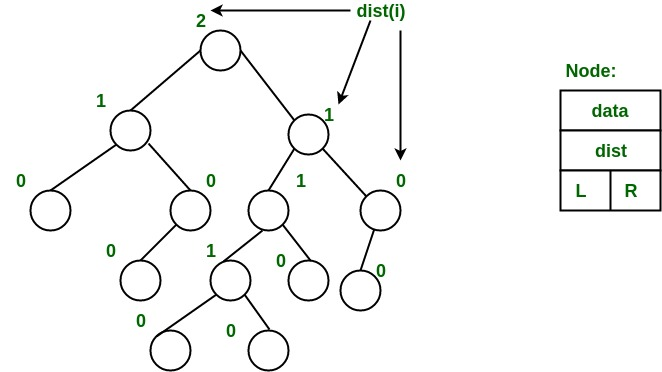From above second property, we can draw two conclusions :

1. The path from root to rightmost leaf is the shortest path from root to a leaf.
2. If the path to rightmost leaf has x nodes, then leftist heap has atleast 2x – 1 nodes. This means the length of path to rightmost leaf is O(log n) for a leftist heap with n nodes.

Operations :

1. The main operation is merge().
2. deleteMin() (or extractMin() can be done by removing root and calling merge() for left and right subtrees.
3. insert() can be done be create a leftist tree with single key (key to be inserted) and calling merge() for given tree and tree with single node.

Idea behind Merging : Since right subtree is smaller, the idea is to merge right subtree of a tree with other tree. Below are abstract steps.

1. Put the root with smaller value as the new root.
2. Hang its left subtree on the left.
3. Recursively merge its right subtree and the other tree.
4. Before returning from recursion: – Update dist() of merged root. – Swap left and right subtrees just below root, if needed, to keep leftist property of merged result

Detailed Steps for Merge:

1. Compare the roots of two heaps.
2. Push the smaller key into an empty stack, and move to the right child of smaller key.
3. Recursively compare two keys and go on pushing the smaller key onto the stack and move to its right child.
4. Repeat until a null node is reached.
5. Take the last node processed and make it the right child of the node at top of the stack, and convert it to leftist heap if the properties of leftist heap are violated.
6. Recursively go on popping the elements from the stack and making them the right child of new stack top.

Example: Consider two leftist heaps given below: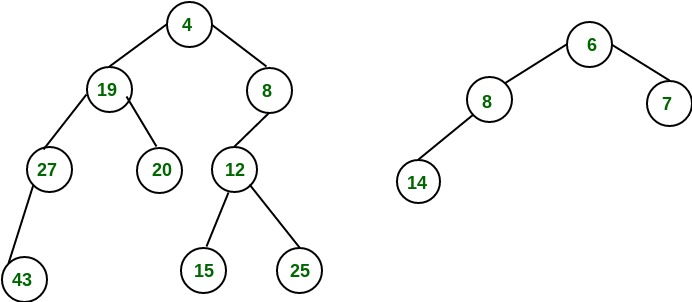Merge them into a single leftist heap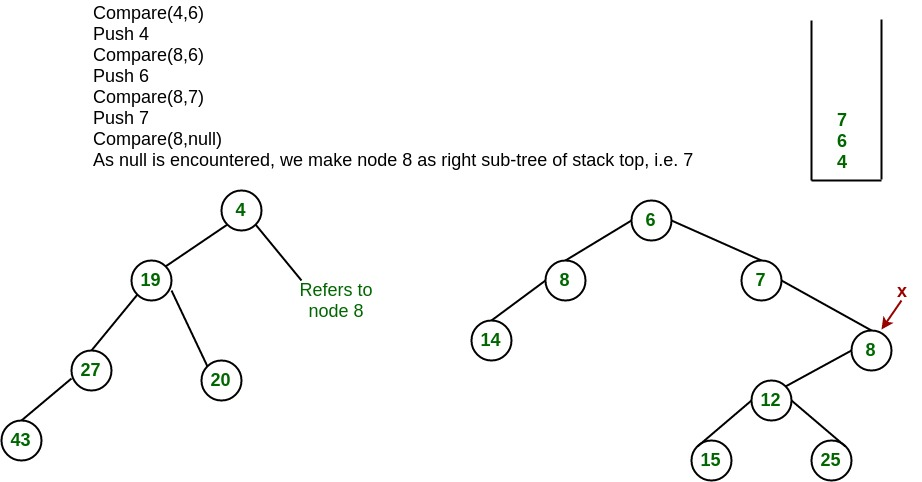The subtree at node 7 violates the property of leftist heap so we swap it with the left child and retain the property of leftist heap.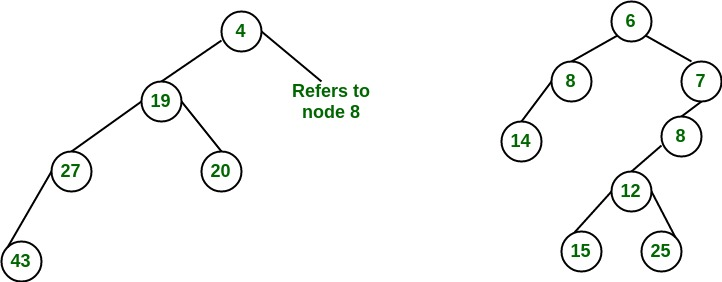Convert to leftist heap. Repeat the process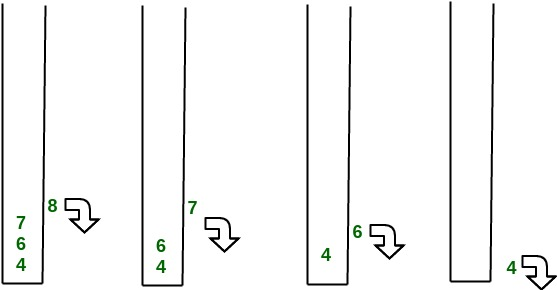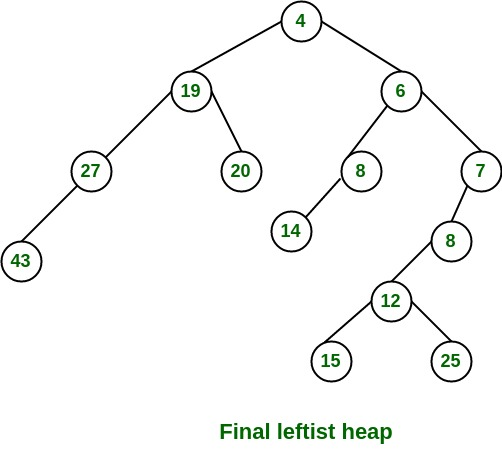The worst case time complexity of this algorithm is O(log n) in the worst case, where n is the number of nodes in the leftist heap. Another example of merging two leftist heap: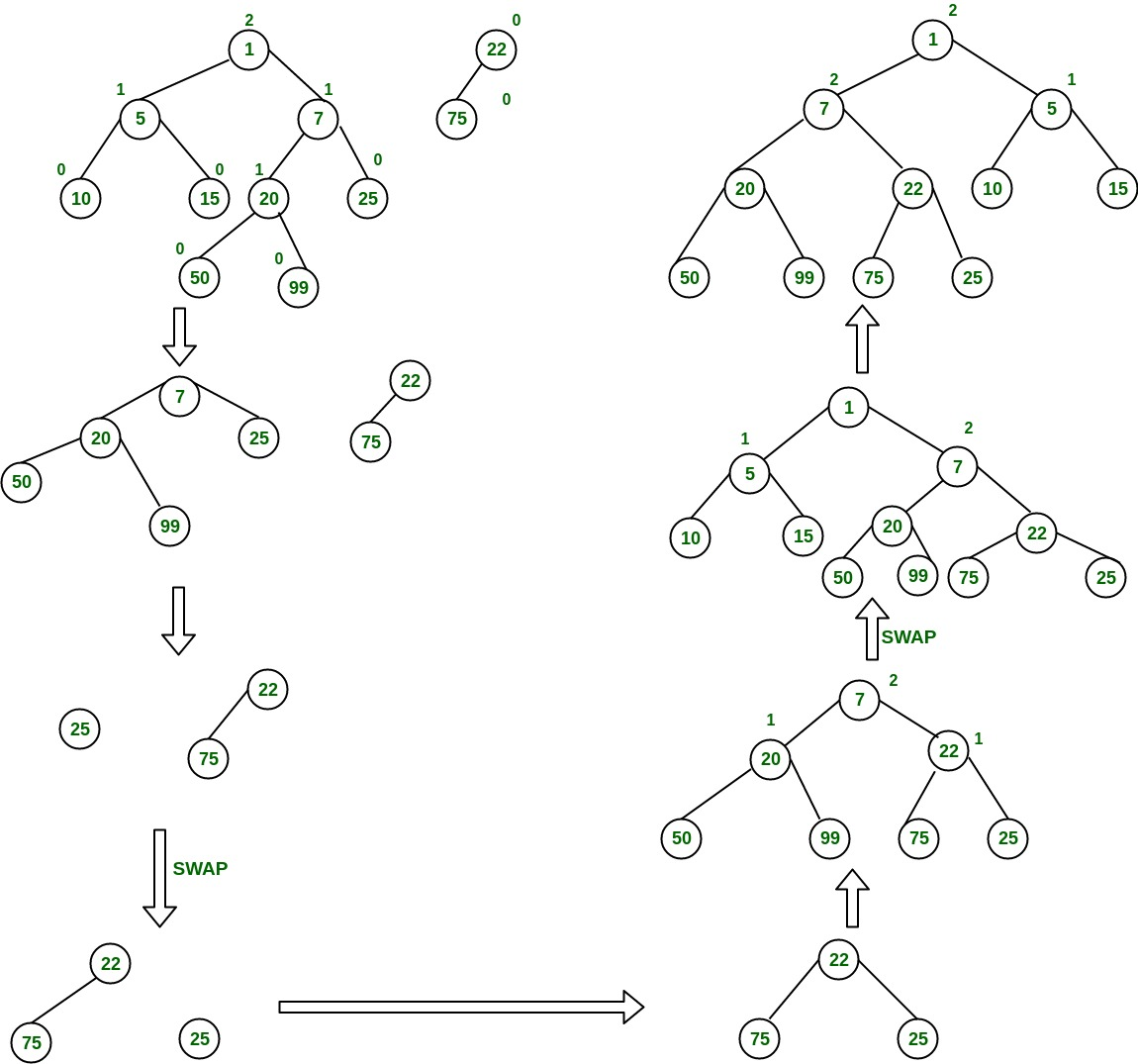Implementation of leftist Tree / leftist Heap:

## CPP

 `//C++ program for leftist heap / leftist tree` `#include ` `using` `namespace` `std;`   `// Node Class Declaration` `class` `LeftistNode` `{` `public``:` `    ``int` `element;` `    ``LeftistNode *left;` `    ``LeftistNode *right;` `    ``int` `dist;` `    ``LeftistNode(``int` `& element, LeftistNode *lt = NULL,` `                ``LeftistNode *rt = NULL, ``int` `np = 0)` `    ``{` `        ``this``->element = element;` `        ``right = rt;` `        ``left = lt,` `        ``dist = np;` `    ``}` `};`   `//Class Declaration` `class` `LeftistHeap` `{` `public``:` `    ``LeftistHeap();` `    ``LeftistHeap(LeftistHeap &rhs);` `    ``~LeftistHeap();` `    ``bool` `isEmpty();` `    ``bool` `isFull();` `    ``int` `&findMin();` `    ``void` `Insert(``int` `&x);` `    ``void` `deleteMin();` `    ``void` `deleteMin(``int` `&minItem);` `    ``void` `makeEmpty();` `    ``void` `Merge(LeftistHeap &rhs);` `    ``LeftistHeap & operator =(LeftistHeap &rhs);` `private``:` `    ``LeftistNode *root;` `    ``LeftistNode *Merge(LeftistNode *h1,` `                    ``LeftistNode *h2);` `    ``LeftistNode *Merge1(LeftistNode *h1,` `                        ``LeftistNode *h2);` `    ``void` `swapChildren(LeftistNode * t);` `    ``void` `reclaimMemory(LeftistNode * t);` `    ``LeftistNode *clone(LeftistNode *t);` `};`   `// Construct the leftist heap` `LeftistHeap::LeftistHeap()` `{` `    ``root = NULL;` `}`   `// Copy constructor.` `LeftistHeap::LeftistHeap(LeftistHeap &rhs)` `{` `    ``root = NULL;` `    ``*``this` `= rhs;` `}`   `// Destruct the leftist heap` `LeftistHeap::~LeftistHeap()` `{` `    ``makeEmpty( );` `}`   `/* Merge rhs into the priority queue.` `rhs becomes empty. rhs must be different` `from this.*/` `void` `LeftistHeap::Merge(LeftistHeap &rhs)` `{` `    ``if` `(``this` `== &rhs)` `        ``return``;` `    ``root = Merge(root, rhs.root);` `    ``rhs.root = NULL;` `}`   `/* Internal method to merge two roots.` `Deals with deviant cases and calls recursive Merge1.*/` `LeftistNode *LeftistHeap::Merge(LeftistNode * h1,` `                                ``LeftistNode * h2)` `{` `    ``if` `(h1 == NULL)` `        ``return` `h2;` `    ``if` `(h2 == NULL)` `        ``return` `h1;` `    ``if` `(h1->element < h2->element)` `        ``return` `Merge1(h1, h2);` `    ``else` `        ``return` `Merge1(h2, h1);` `}`   `/* Internal method to merge two roots.` `Assumes trees are not empty, and h1's root contains` `smallest item.*/` `LeftistNode *LeftistHeap::Merge1(LeftistNode * h1,` `                                ``LeftistNode * h2)` `{` `    ``if` `(h1->left == NULL)` `        ``h1->left = h2;` `    ``else` `    ``{` `        ``h1->right = Merge(h1->right, h2);` `        ``if` `(h1->left->dist < h1->right->dist)` `            ``swapChildren(h1);` `        ``h1->dist = h1->right->dist + 1;` `    ``}` `    ``return` `h1;` `}`   `// Swaps t's two children.` `void` `LeftistHeap::swapChildren(LeftistNode * t)` `{` `    ``LeftistNode *tmp = t->left;` `    ``t->left = t->right;` `    ``t->right = tmp;` `}`   `/* Insert item x into the priority queue, maintaining` `heap order.*/` `void` `LeftistHeap::Insert(``int` `&x)` `{` `    ``root = Merge(``new` `LeftistNode(x), root);` `}`   `/* Find the smallest item in the priority queue.` `Return the smallest item, or throw Underflow if empty.*/` `int` `&LeftistHeap::findMin()` `{` `    ``return` `root->element;` `}`   `/* Remove the smallest item from the priority queue.` `Throws Underflow if empty.*/` `void` `LeftistHeap::deleteMin()` `{` `    ``LeftistNode *oldRoot = root;` `    ``root = Merge(root->left, root->right);` `    ``delete` `oldRoot;` `}`   `/* Remove the smallest item from the priority queue.` `Pass back the smallest item, or throw Underflow if empty.*/` `void` `LeftistHeap::deleteMin(``int` `&minItem)` `{` `    ``if` `(isEmpty())` `    ``{` `        ``cout<<``"Heap is Empty"``<left);` `        ``reclaimMemory(t->right);` `        ``delete` `t;` `    ``}` `}`   `// Internal method to clone subtree.` `LeftistNode *LeftistHeap::clone(LeftistNode * t)` `{` `    ``if` `(t == NULL)` `        ``return` `NULL;` `    ``else` `        ``return` `new` `LeftistNode(t->element, clone(t->left),` `                            ``clone(t->right), t->dist);` `}`   `//Driver program` `int` `main()` `{` `    ``LeftistHeap h;` `    ``LeftistHeap h1;` `    ``LeftistHeap h2;` `    ``int` `x;` `    ``int` `arr[]= {1, 5, 7, 10, 15};` `    ``int` `arr1[]= {22, 75};`   `    ``h.Insert(arr);` `    ``h.Insert(arr);` `    ``h.Insert(arr);` `    ``h.Insert(arr);` `    ``h.Insert(arr);` `    ``h1.Insert(arr1);` `    ``h1.Insert(arr1);`   `    ``h.deleteMin(x);` `    ``cout<< x <

Output

```1
22
5```

Time Complexity: The time complexity of all operations like Insert(), deleteMin(), findMin() and Merge() on a Leftist Heap is O(log n). This is because the height of a Leftist Heap is always O(log n).

Auxiliary Space: The space complexity of a Leftist Heap is O(n). This is because the Leftist Heap requires space for storing n number of elements.

1. Efficient extract-min operation: The extract-min operation has a time complexity of O(log n), making it one of the most efficient data structures for this operation.
2. Efficient merging: The merge operation has a time complexity of O(log n), making it one of the fastest data structures for merging two binary heaps.
3. Simple implementation: The leftist tree has a relatively simple implementation compared to other binary heap data structures, such as Fibonacci heaps.

1. Slower insert operation: The insert operation in a leftist tree has a time complexity of O(log n), making it slower than other binary heap data structures, such as binary heaps.
2. Increased memory usage: The leftist tree uses more memory than other binary heap data structures, such as binary heaps, due to its requirement for the maintenance of null path length values for each node.

### References and books:

1. “Data Structures and Algorithm Analysis in Java” by Mark Allen Weiss.
2. “Introduction to Algorithms” by Thomas H. Cormen, Charles E. Leiserson, Ronald L. Rivest, and Clifford Stein.
3. “Purely functional data structures” by Chris Okasaki.
4. “The Art of Computer Programming, Volume 1: Fundamental Algorithms” by Donald E. Knuth.

This article is contributed by Sheena Kohli and Minal Sunil Parchand. If you like GeeksforGeeks and would like to contribute, you can also write an article using write.geeksforgeeks.org or mail your article to review-team@geeksforgeeks.org. See your article appearing on the GeeksforGeeks main page and help other Geeks.

My Personal Notes arrow_drop_up